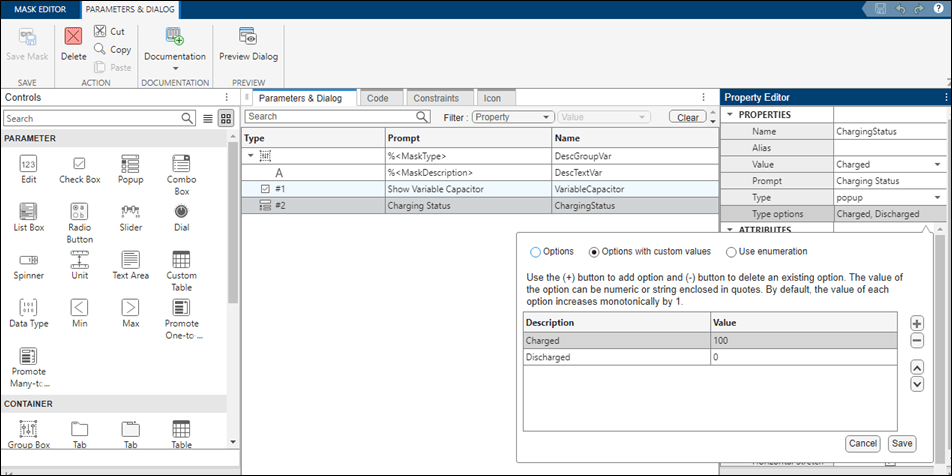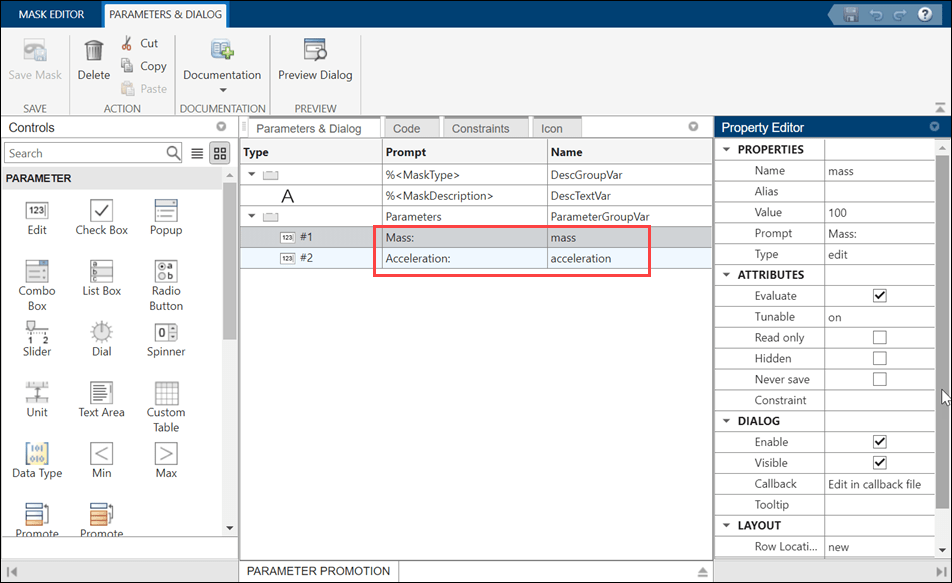# Preserve Tunability of Parameters That Are Modified or Created in Mask Initialization

A desirable feature of code generated from a model is the ability to tune variables to different values. To achieve this, user-defined variables in the model must be present in the generated code. If a model contained blocks with mask initialization commands that creates a new variable or modified an existing mask dialog parameter in the mask initialization, and if that parameter or variable is referenced in a child block, the values of the mask parameter or variable were in-lined in the generated code. The parameters were not tunable even if they referred to a workspace variable.

You can preserve the tunability of the mask parameters. The expressions corresponding to mask parameters referenced in the child block appear in the generated code. The expression is generated even if the parameters are created in mask initialization section of the top-level mask. The tunability of these parameters is retained only if the mask initialization code is created using a mask callback file and the parameter value is defined in the format mentioned in the example. See Organize Mask Initialization and Callbacks in a MATLAB File.

### Explore the Model

This example shows two scenarios in which the tunability of parameters is preserved in the generated code.

The first scenario demonstrates how tunability is preserved when a parameter is modified in the mask initialization code. The model contains a Subsystem block `DataRounding`, which rounds numerical data by a preferred rounding method. The value of the mask parameter is modified in the mask initialization code according to the selected rounding method in mask dialog box.

The second scenario demonstrates how tunability is preserved when a parameter is created in the mask initialization code. The model contains a Subsystem block `CalculateForce` which calculates force as the product of mass and acceleration. The parameter `outForce` is created in the mask initialization code to calculate force.

```open_system("slexMaskPreserveTunabilityOfMaskInitVariable.slx") ```Preserve the tunability of a parameter in generated code if the mask parameter is modified in the mask initialization code.

1. Create a mask on the Subsystem block `DataRounding`.

2. In the Mask Editor, create an edit parameter `Data` and a popup parameter `DataRoundingMode` with options `floor`, `Round`, and `Nearest`.3. In the Code tab, enter the Callback File Name as `maskInitFuncDataChange`. Include this code in the editor.

```classdef maskInitFuncDataChange methods(Static) function MaskInitialization(maskInitContext) ws = maskInitContext.MaskWorkspace; ws.set('Data','maskInitFuncDataChange.updateData','Data', 'DataRoundingMode'); end function outData = updateData(inData, inRoundingMode) switch(inRoundingMode) case 1 outData = floor(inData); case 2 outData = round(inData); case 3 outData = nearest(inData); otherwise outData = inRoundingMode; end end end end ```

The function `updateData` updates the parameter `Data` based on the selected `DataRoundingMode`.

5. Double-click the block `DataRounding`. In the Block Parameters dialog box, enter the value for Data as `5.3` and select the Data Rounding Mode as `Round`. Click OK.

6. Simulate the model and generate code.

```/* Model step function */ void slexMaskPreserveTunabilityOfMaskInitVariable_step(void) { /* Outport: '<Root>/Out1' incorporates: * Constant: '<S1>/Constant' */ switch ((int32_T)slexMaskPreserveTunabilityOfM_P.DataRounding_DataRoundingMode) { case 1: slexMaskPreserveTunabilityOfM_Y.Out1 = slexMaskPreserveTunabilityOfM_P.DataRounding_Data; slexMaskPreserveTunabilityOfM_Y.Out1 = floor (slexMaskPreserveTunabilityOfM_Y.Out1); break; case 2: slexMaskPreserveTunabilityOfM_Y.Out1 = slexMaskPreserveTunabilityOfM_P.DataRounding_Data; slexMaskPreserveTunabilityOfM_Y.Out1 = rt_roundd_snf (slexMaskPreserveTunabilityOfM_Y.Out1); break; case 3: slexMaskPreserveTunabilityOfM_Y.Out1 = slexMaskPreserveTunabilityOfM_P.DataRounding_Data + 0.5; slexMaskPreserveTunabilityOfM_Y.Out1 = floor (slexMaskPreserveTunabilityOfM_Y.Out1); break; default: slexMaskPreserveTunabilityOfM_Y.Out1 = slexMaskPreserveTunabilityOfM_P.DataRounding_DataRoundingMode; break; } ```
``` /* End of Outport: '<Root>/Out1' */ }```

Observe that tunability is preserved for the mask parameter `Data` in the generated code.

In the example model, the subsystem block `CalculateForce` calculates force. The parameter `outForce` is created in the mask initialization code to calculate force as the product of mass and acceleration. The generated code contains an expression for the parameter `outForce`.

1. Create a mask on the subsystem `CalculateForce`.

2. In the Mask Editor, create edit parameters `mass` and `acceleration`.3. In the Code tab, enter the Callback File Name as `maskInitFuncNewVariable`. Include this in the editor.

```classdef maskInitFuncNewVariable methods(Static) function MaskInitialization(maskInitContext) ws = maskInitContext.MaskWorkspace; ws.set('force','maskInitFuncNewVariable.calculateForce', 'mass', 'acceleration'); end function outForce = calculateForce(inMass, inAcceleration) outForce = inMass * inAcceleration; end end end ```

The function `calculateForce` takes the arguments `mass` and `acceleration` and calculates the `outForce` as the product of `mass` and `acceleration`. See Author Mask Callback Code in MATLAB File section in Organize Mask Initialization and Callbacks in a MATLAB File for more information about setting and retrieving values from mask workspace.

5. Double-click the block `CalculateForce`. In the Block Parameters dialog box, enter the value for Mass as `100` and Acceleration as `10`. Click OK.

6. Simulate the model and generate code.

```/* Model step function */ void slexMaskPreserveTunabilityOfMaskInitVariable_step(void) { /* Outport: '<Root>/Out2' incorporates: * Constant: '<S1>/Constant' */ slexMaskPreserveTunabilityOfM_Y.Out2 = slexMaskPreserveTunabilityOfM_P.CalculateForce_mass * slexMaskPreserveTunabilityOfM_P.CalculateForce_acceleration; } ```

Observe that the expression corresponding to the variable is generated in the code.

### Limitations

• Tunability will not be preserved if the function that modifies or creates the mask parameter contains Simulink® APIs such as `get_param` and `set_param`.

• Tunability will not be preserved if the same mask parameter is modified using a simple set API(ws.set('Parameter1', '5'). Tunability of the mask parameter is retained only if the variable is modified using ws.set(' `modified/new variableName` ', ' `outpuitFunction` ', ' `maskParamName1` ', ' `maskParamName2` ') format.

• To preserve tunability, write any such code involving get_param and set_param inside the mask initialization function and pass the value as an argument to the function which modifies/creates mask parameter value.

• The function call to modify or create mask parameters must be in the following format:

i. The first argument of the function call must be the mask parameter that you want to modify or create. This argument must be a string.

ii. The second argument must be the function that modifies or creates the mask parameter and it should be in the form `className`. `functionName`. The function must be static.

iii. The next set of arguments must be mask parameter names that are passed to the static function. These parameters must be strings.

iv. Other values you want to pass to this function must not be strings. You must pass the argument names or values.

Example:

```classdef mycallback methods(Static) function MaskInitialization(maskInitContext) ws = maskInitContext.MaskWorkspace; constVal = 5; ws.set('newVar','mycallback.update','maskParam1', constVal); end function out = update(in1, in2) out = in1+in2; end end end ```